### Installation

You can install the development version from GitHub with:

# install.packages("remotes")
remotes::install_github("yjunechoe/ggtrace")

library(ggtrace)

More on the 📦 package website: https://yjunechoe.github.io/ggtrace

### Description

ggtrace embodies an opinionated approach to learning/debugging/hacking ggplot2 internals. I recommend watching the following presentation(s) on ggtrace before getting started on any kind of code:

You can read the full philosophy behind ggtrace in the Getting Started vignette. But broadly speaking, ggtrace was designed with three goals in mind, in order of increasing complexity:

1. To help users understand the design of sublayer modularity and write more expressive layer code using delayed aesthetic evaluation. This is a primarily pedagogical goal and outlined in my paper Sub-layer modularity in the Grammar of Graphics. The family of layer_*() extractor functions return snapshots of layer data in the internals, to help scaffold a mental model of sublayer processes as a data wrangling pipeline.2. To facilitate the user-developer transition, empowering experienced users of ggplot2 to start developing their own extension packages. This is achieved via a family of inspect, capture, and highjack workflow functions, which provide a functional interface into the object-oriented design of the internals (the <ggproto> OOP).3. To provide a pseudo-extension mechanism for ggplot2, by injecting custom code that highjacks the rendering pipeline. This is similar in spirit to {gggrid} and {gginnards}, but with a broader scope (targeting any arbitrary computation) at some cost to reproducibility (may break with even trivial changes to the ggplot2 codebase). This is achieved via the low-level function ggtrace() (which improves upon base::trace()) and its functional form with_ggtrace(). See examples in the Overview vignette.

## Example usage

library(ggplot2)
packageVersion("ggplot2")
#>  '3.4.3.9000'

### 1) Inspect sub-layer data

Example adopted from Demystifying delayed aesthetic evaluation

A bar plot of counts with geom_bar() with stat = "count" default:

bar_plot <- ggplot(mpg, aes(class, fill = class)) +
geom_bar() +
theme(legend.position = "none")

State of bar layer’s data after the statistical transformation step:

ggtrace::layer_after_stat(bar_plot)
#> ✔ Executed ggtrace_inspect_return(bar_plot, ggplot2:::Layer$compute_statistic) #> # A tibble: 7 × 8 #> count prop x width flipped_aes fill PANEL group #> <dbl> <dbl> <mppd_dsc> <dbl> <lgl> <chr> <fct> <int> #> 1 5 1 1 0.9 FALSE 2seater 1 1 #> 2 47 1 2 0.9 FALSE compact 1 2 #> 3 41 1 3 0.9 FALSE midsize 1 3 #> 4 11 1 4 0.9 FALSE minivan 1 4 #> 5 33 1 5 0.9 FALSE pickup 1 5 #> 6 35 1 6 0.9 FALSE subcompact 1 6 #> 7 62 1 7 0.9 FALSE suv 1 7 We can map aesthetics to variables from the after-stat data using after_stat(): bar_plot + geom_text( aes(label = after_stat(count)), stat = "count", position = position_nudge(y = 1), vjust = 0 )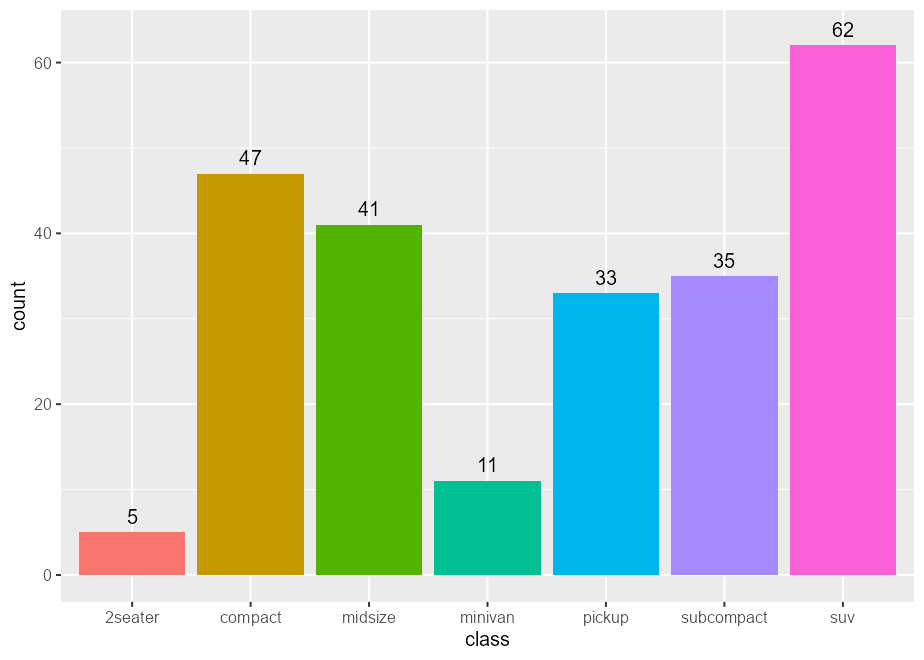Same idea with after_scale(): scatter_plot <- ggplot(mpg, aes(displ, hwy, fill = class)) + scale_fill_viridis_d(option = "magma") scatter_plot + geom_point(shape = 21, size = 4, stroke = 1)# fill column available for after_scale(fill) ggtrace::layer_after_scale(scatter_plot) #> ✔ Executed ggtrace_inspect_return(scatter_plot, ggplot2:::Layer$compute_geom_2)
#> # A tibble: 234 × 5
#>    fill          x     y PANEL group
#>    <chr>     <dbl> <dbl> <fct> <int>
#>  1 #2D1160FF   1.8    29 1         2
#>  2 #2D1160FF   1.8    29 1         2
#>  3 #2D1160FF   2      31 1         2
#>  4 #2D1160FF   2      30 1         2
#>  5 #2D1160FF   2.8    26 1         2
#>  6 #2D1160FF   2.8    26 1         2
#>  7 #2D1160FF   3.1    27 1         2
#>  8 #2D1160FF   1.8    26 1         2
#>  9 #2D1160FF   1.8    25 1         2
#> 10 #2D1160FF   2      28 1         2
#> # ℹ 224 more rows

scatter_plot +
geom_point(
aes(color = after_scale(prismatic::best_contrast(fill))),
shape = 21, size = 4, stroke = 1
)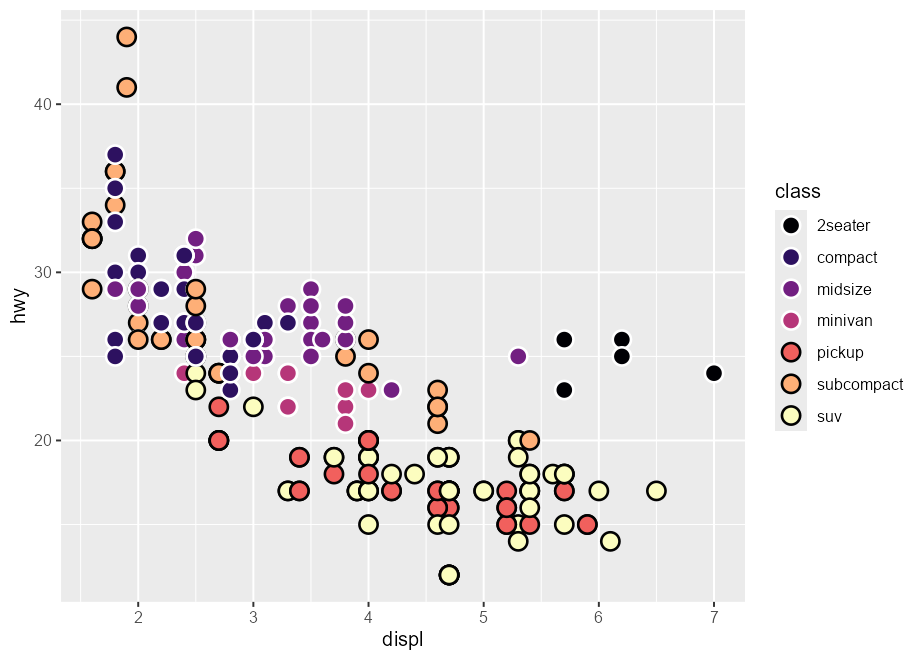### 2) Debug sublayer data

Example adopted from my rstudio::conf 2022 talk

Given a boxplot made with a geom_boxplot() layer, suppose that we want to add a second layer annotating the value of the upper whiskers:

box_p <- ggplot(data = mtcars) +
aes(x = factor(cyl), y = mpg) +
geom_boxplot()
box_p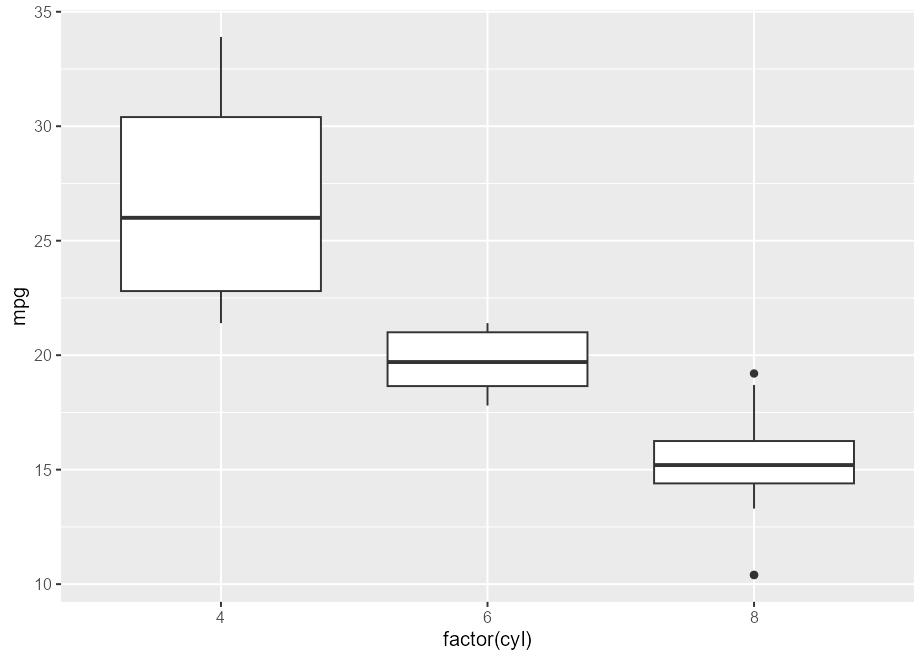A naive approach would be to add a layer that combines a boxplot stat with a label geom. But this errors out of the box:

box_p +
geom_label(stat = "boxplot")
#> Error in geom_label():
#> ! Problem while setting up geom.
#> ℹ Error occurred in the 2nd layer.
#> Caused by error in compute_geom_1():
#> ! geom_label() requires the following missing aesthetics: y and label

The error tells us that the geom is missing some missing aesthetics, so something must be wrong with the data that the geom receives. If we inspect this using layer_before_geom(), we find that the columns for y and label are indeed missing in the Before Geom data:

layer_before_geom(last_plot(), layer = 2L, error = TRUE, verbose = FALSE)
#> # A tibble: 3 × 14
#>    ymin lower middle upper  ymax outliers  notchupper notchlower     x width
#>   <dbl> <dbl>  <dbl> <dbl> <dbl> <list>         <dbl>      <dbl> <dbl> <dbl>
#> 1  21.4  22.8   26    30.4  33.9 <dbl >       29.6       22.4     1  0.75
#> 2  17.8  18.6   19.7  21    21.4 <dbl >       21.1       18.3     2  0.75
#> 3  13.3  14.4   15.2  16.2  18.7 <dbl >       16.0       14.4     3  0.75
#> # ℹ 4 more variables: relvarwidth <dbl>, flipped_aes <lgl>, PANEL <fct>,
#> #   group <int>

Thus, we need to ensure that y exists to satisfy both the stat and the geom, and that label exists after the statistical transformation step but before the geom sees the data. Crucially, we use the computed variable ymax to (re-)map to the y and label aesthetics.

box_p +
geom_label(
aes(y = stage(mpg, after_stat = ymax),
label = after_stat(ymax)),
stat = "boxplot"
)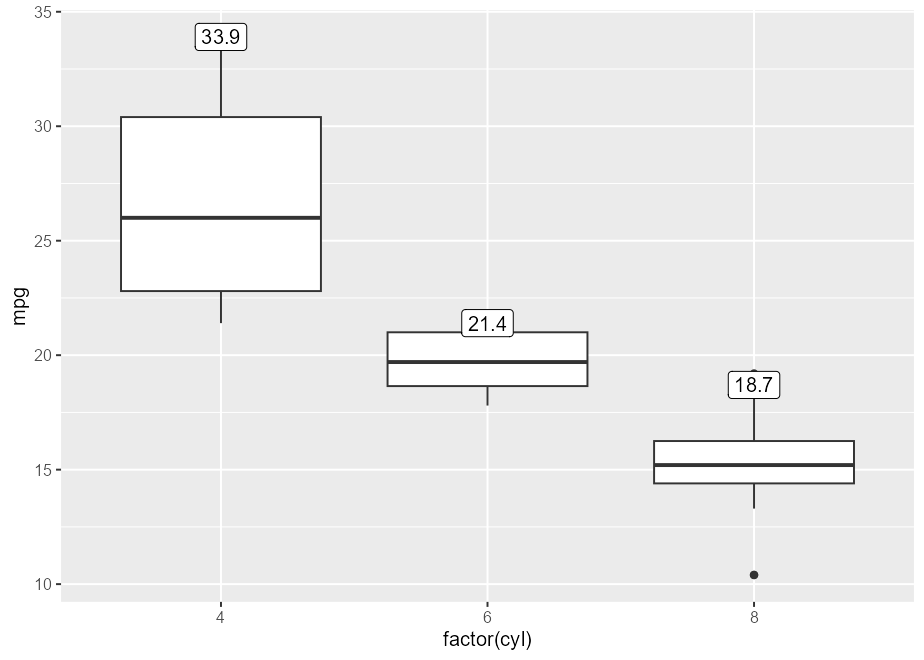### 3) Highjack ggproto (remove boxplot outliers)

Example inspired by https://github.com/tidyverse/ggplot2/issues/4892.

You can hide outliers in geom_boxplot() using the outlier. argument(s), but they’ll still be present in the layer’s underlying dataframe representation. Note how this method adds empty space around the boxplot:

boxplot_plot <- ggplot(mpg, aes(hwy, class)) +
geom_boxplot(outlier.shape = NA)
boxplot_plot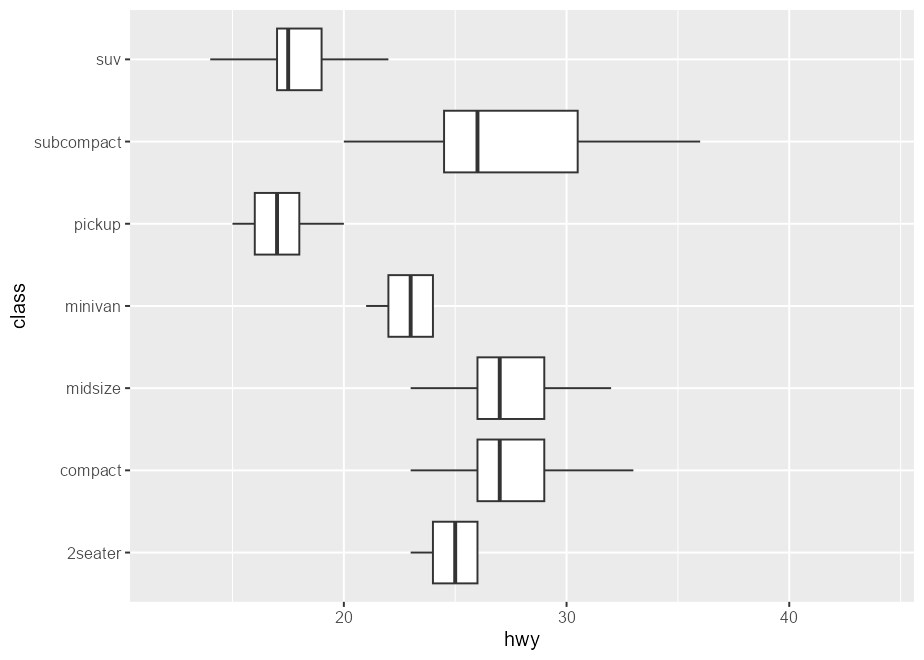This is because the scales are re-trained after the calculation of the boxplot statistics. In other words, the “final” min/max value of the x-scale are derived from the calculated outliers, even if they’re not drawn.

layer_data(boxplot_plot)[, c("xmin", "xmax", "outliers", "xmin_final", "xmax_final")]
#>   xmin xmax                       outliers xmin_final xmax_final
#> 1   23   26                                        23         26
#> 2   23   33                 35, 37, 35, 44         23         44
#> 3   23   32                                        23         32
#> 4   21   24                             17         17         24
#> 5   15   20                 12, 12, 12, 22         12         22
#> 6   20   36                         44, 41         20         44
#> 7   14   22 12, 12, 25, 24, 27, 25, 26, 23         12         27

One solution is to highjack the calculation of the boxplot layer’s statistics such that values of the outliers column is set to NULL. In ggtrace_highjack_return(), we pass an expression that modifies returnValue() to the value argument, which evaluates to the value about to be returned by the method.

ggtrace_highjack_return(
x = boxplot_plot,
method = Stat$compute_layer, cond = 1L, value = quote({ transform(returnValue(), outliers = NULL) }) )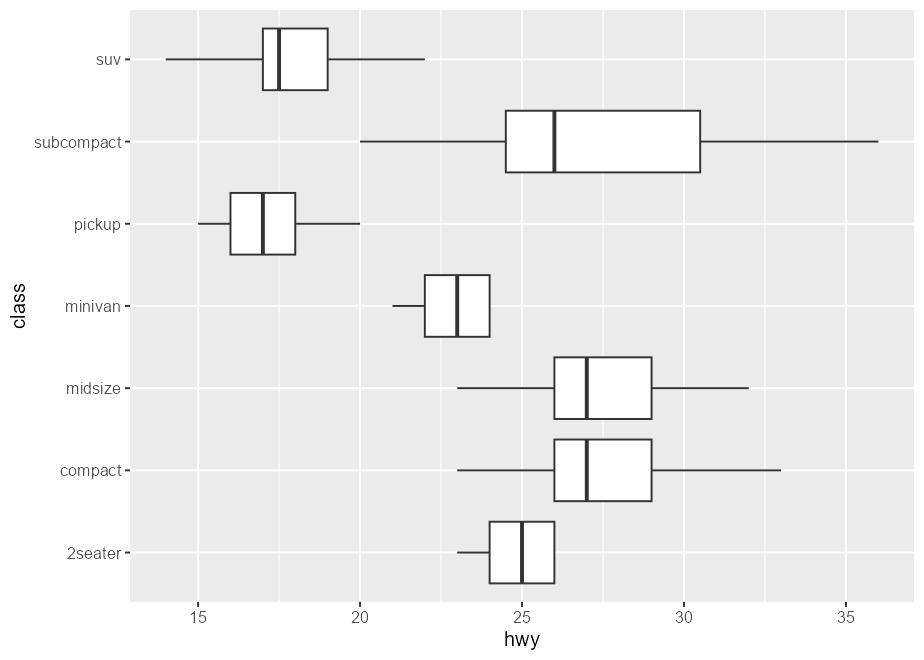Note that this is also possible in “vanilla” ggplot. Following our earlier discussion of after_stat(): # Suppress warning from mapping to outliers aesthetic update_geom_defaults("boxplot", list(outliers = NULL)) ggplot(mpg, aes(hwy, class)) + geom_boxplot( # Equivalent effect of modifying the after-stat data aes(outliers = after_stat(list(NULL))) )### 4) Not just ggproto Example adopted from Github issue #97 The method argument of ggtrace_*() workflow functions can be (almost) any function-like object called during the rendering of a ggplot. set.seed(2023) # Example from ?stat_summary summary_plot <- ggplot(mtcars, aes(mpg, factor(cyl))) + geom_point() + stat_summary(fun.data = "mean_cl_boot", colour = "red", linewidth = 2, size = 3) summary_plot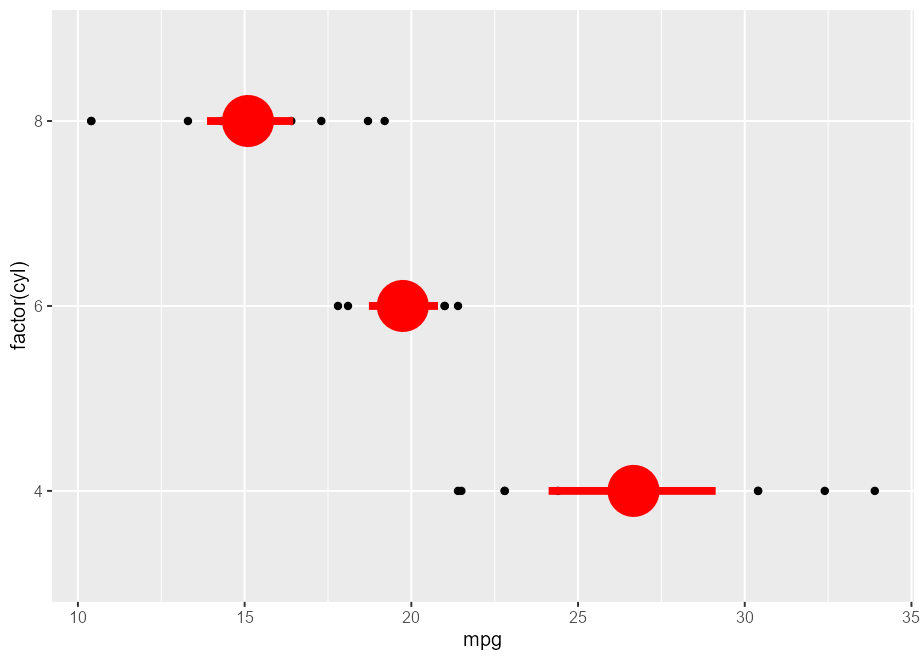ggtrace_inspect_args(x = summary_plot, method = mean_cl_boot) #>$x
#>   22.8 24.4 22.8 32.4 30.4 33.9 21.5 27.3 26.0 30.4 21.4
ggtrace_inspect_return(x = summary_plot, method = mean_cl_boot)
#>          y     ymin     ymax
#> 1 26.66364 24.11727 29.19159

ggtrace_highjack_return(
x = summary_plot, method = mean_cl_boot,
value = quote({
data.frame(y = 50, ymin = 25, ymax = 75)
})
)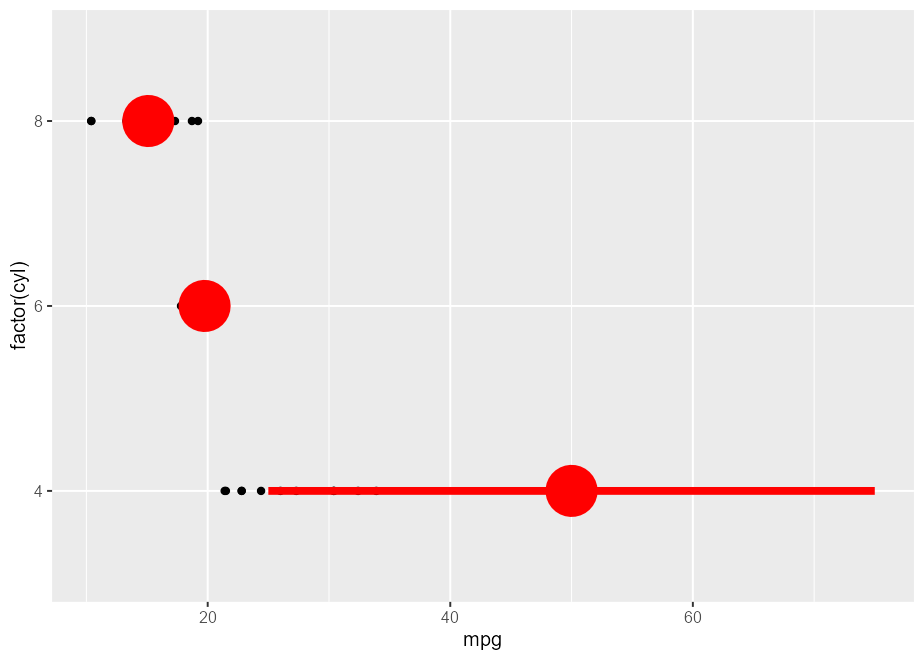### 5) Visually crop polar plots

Here’s a plot in polar coordinates:

polar_plot <- ggplot(mtcars, aes(hp, mpg)) +
geom_point() +
geom_smooth(method = "lm", formula = y ~ x) +
expand_limits(y = c(0, 60)) +
coord_polar(start = 0, theta = "y")

polar_plot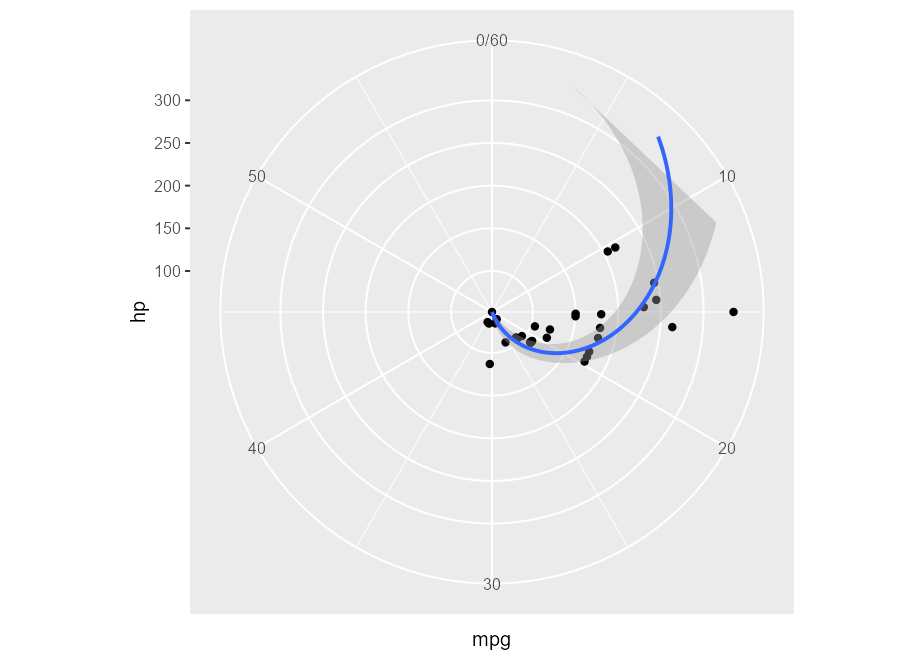We can clip the plot panel by highjacking the Layout$render() method using the generic workflow function with_ggtrace(): with_ggtrace( x = polar_plot + theme(aspect.ratio = 1/.48), method = Layout$render,
trace_steps = 5L,
trace_expr = quote({
panels[] <- editGrob(panels[], vp = viewport(xscale = c(.48, 1)))
}),
out = "g"
)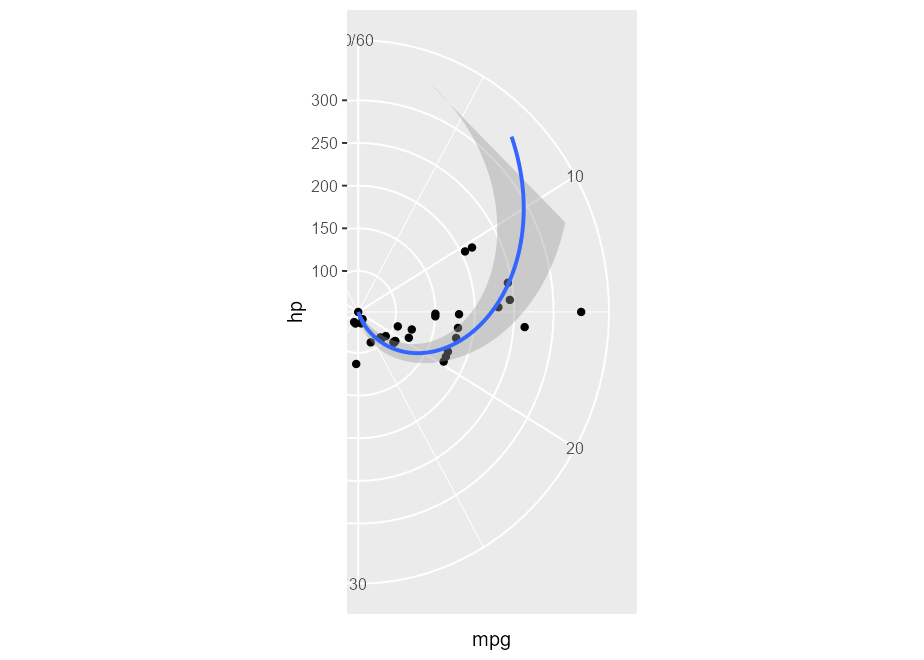See implementation in MSBMisc::crop_coord_polar().

### 6) Highjack the internal data pipeline

Example inspired by a stackoverflow question:

bars <- ggplot(mpg, aes(class)) +
geom_bar(aes(fill = drv), color = "grey")
bars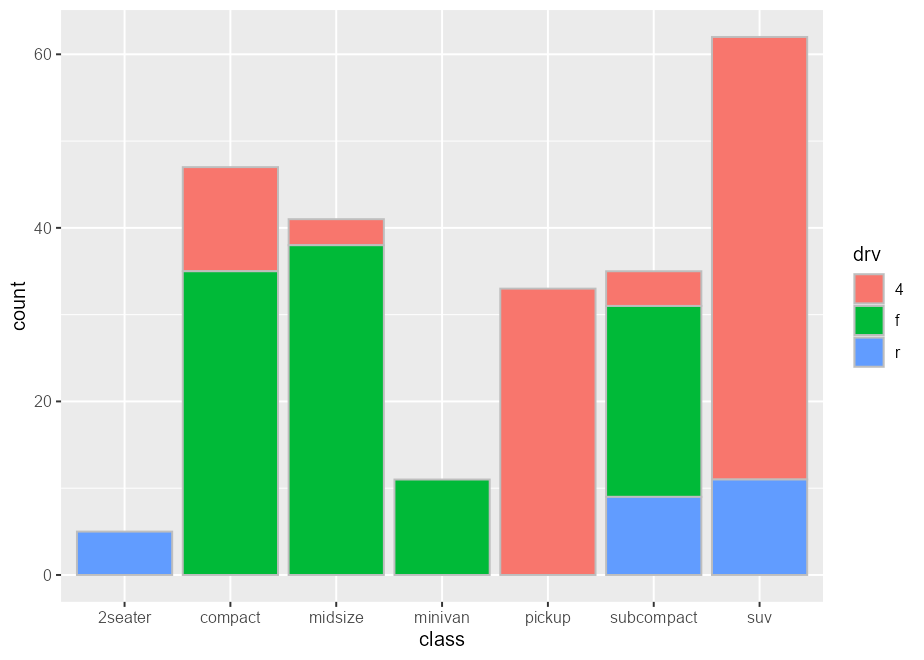Intercepting the data at draw step to subset bars arbitrarily:

bars_subset <- ggtrace_highjack_args(
x = bars, method = Geom$draw_layer, cond = 1L, values = expression( data = data[c(2, 4, 6, 8, 11),] ) ) bars_subset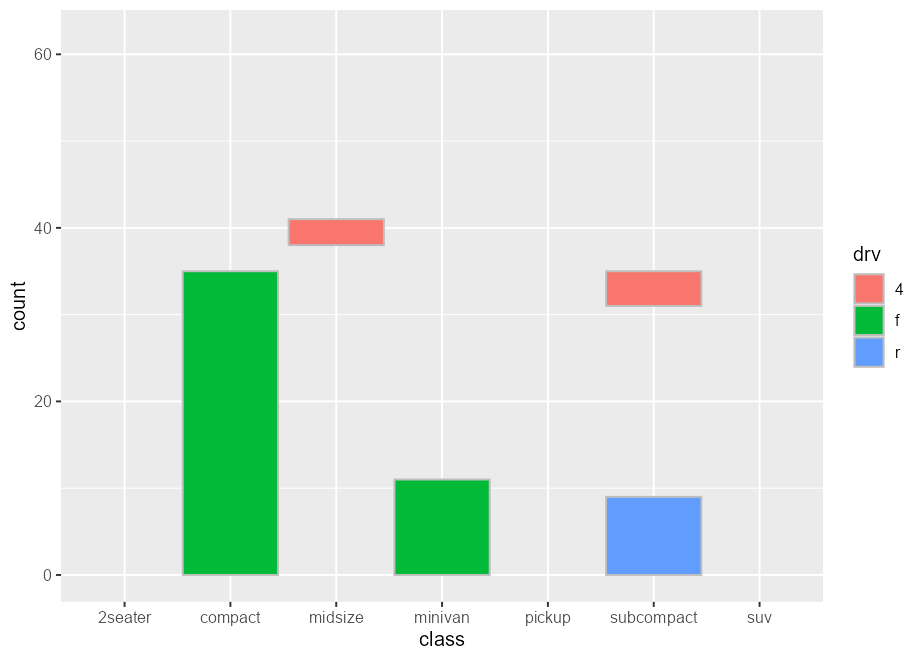### 7) Highjack the internal drawing context Example adopted from my useR! 2022 talk: library(palmerpenguins) flashy_plot <- na.omit(palmerpenguins::penguins) |> ggplot(aes(x = species, y = flipper_length_mm)) + geom_boxplot(aes(fill = species), width = .7) + facet_wrap(~ year) flashy_plotggtrace_highjack_return( flashy_plot, Geom$draw_panel, cond = TRUE,
value = quote({
circ <- circleGrob(y = .25 * ._counter_)
grobTree( editGrob(circ, gp = gpar(fill = linearGradient())),
editGrob(returnValue(), vp = viewport(clip = circ)) )
}))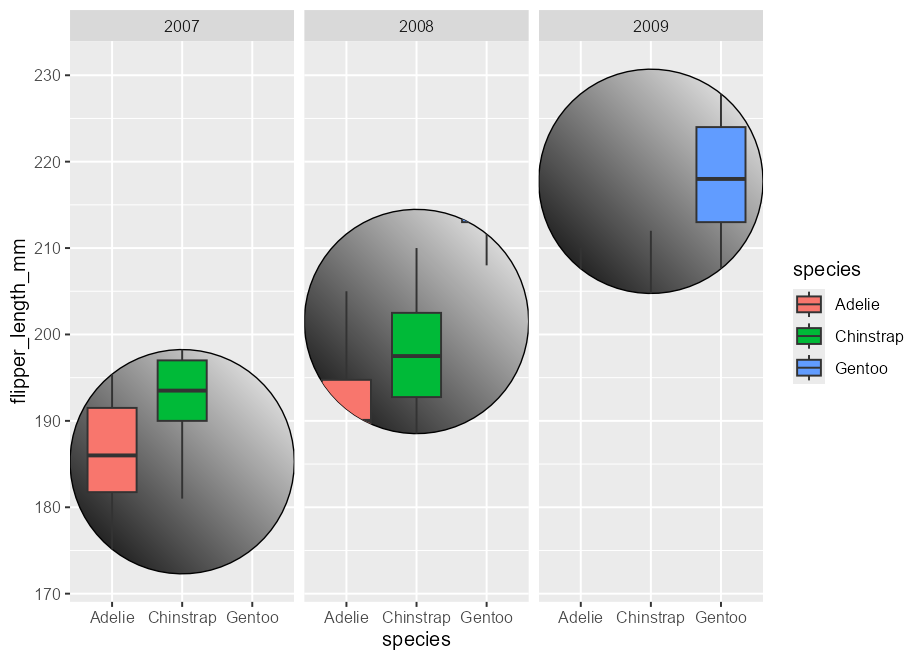Note the use of the special variable ._counter_, which increments every time a function/method has been called. See the tracing context topic for more details.